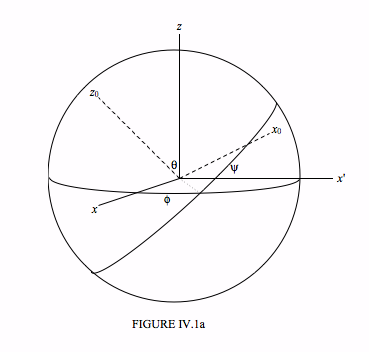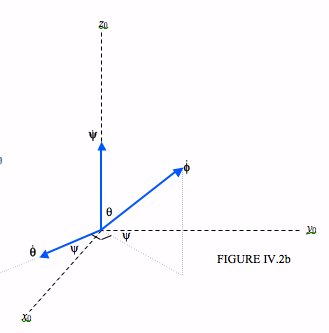$$\require{cancel}$$

4.2: Angular Velocity and Eulerian Angles

•• Contributed by Jeremy Tatum
• Emeritus Professor (Physics & Astronomy) at University of Victoria

Let $$Oxyz$$ be a set of space-fixed axis, and let $$Ox_{0}y_{0}z_{0}$$ be the body-fixed principal axes of a rigid body.The orientation of the body-fixed principal axes $$Ox_{0}y_{0}z_{0}$$ with respect to the space-fixed axes $$Oxyz$$ can be described by the three Euler angles: $$\theta$$, $$\phi$$, and $$\psi$$. These are illustrated in Figure IV.1a. Those who are not familiar with Euler angles or who would like a reminder can refer to their detailed description in Chapter 3 of my notes on Celestial Mechanics.

We are going to examine the motion of a body that is rotating about a non-principal axis. If the body is freely rotating in space with no external torques acting upon it, its angular momentum $$\bf{L}$$ will be constant in magnitude and direction. The angular velocity vector $$\bf{\omega}$$, however, will not be constant, but will wander with respect to both the space-fixed and body-fixed axes, and we shall be examining this motion. I am going to call the instantaneous components of $$\omega$$ relative to the body-fixed axes $$\omega_{1} , \omega_{2}, \omega_{3}$$, and its magnitude $$\omega$$. As the body tumbles over and over, its Euler angles will be changing continuously. We are going to establish a geometrical relation between the instantaneous rates of change of the Euler angles and the instantaneous components of $$\omega$$. That is, we are going to find how $$\omega_{1} , \omega_{2}$$ and $$\omega_{3}$$ are related to $$\dot{\theta} , \dot{\phi}$$ and $$\dot{\psi}$$.

I have indicated, in Figure VI.2a, the angular velocities $$\boldsymbol{\dot{\theta}} , \boldsymbol{\dot{\phi}}$$ and $$\boldsymbol{\dot{\psi}}$$ as vectors in what I hope will be agreed are the appropriate directions.It should be clear that $$\omega_1$$ is equal to the $$x_{0}$$-component of $$\boldsymbol{\dot{\phi}}$$ plus the $$x_{0}$$-component of $$\boldsymbol{\dot{\theta}}$$ and that $$\omega_2$$ is equal to the $$y_{0}$$-component of $$\boldsymbol{\dot{\phi}}$$ plus the $$y_{0}$$-component of $$\boldsymbol{\dot{\theta}}$$ and that $$\omega_3$$ is equal to the $$z_{0}$$-component of $$\boldsymbol{\dot{\phi}}$$ plus $$\boldsymbol{\dot{\psi}}$$

Let us look at Figure IV.2bWe see that the $$x_{0}$$ and $$y_{0}$$ components of $$\boldsymbol{\dot{\theta}}$$ are $$\dot{\theta} \cos \psi$$ and $$- \dot{\theta} \sin \psi$$ respectively. The $$x_{0}$$, $$y_{0}$$ and $$z_{0}$$ components of $$\boldsymbol{\dot{\phi}}$$ are, respectively:

• $$\dot{\phi} \sin \theta \sin \psi$$,
• $$\dot{\phi} \sin \theta \sin \psi$$, and
• $$\dot{\phi} \cos \theta$$.

Hence we arrive at

$\omega_{1} = \dot{\phi} \sin \theta \sin \psi + \dot{\theta} \cos \psi . \tag{4.2.1}\label{eq:4.2.1}$

$\omega_{2} = \dot{\phi} \sin \theta \cos \psi - \dot{\theta} \sin \psi . \tag{4.2.2}\label{eq:4.2.2}$

$\omega_{3} = \dot{\phi} \cos \theta + \dot{ \psi} \tag{4.2.3}\label{eq:4.2.3}$